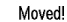# \$B%.%c%i%j!<(B

\$B\$3\$l\$i\$N2hA|\$O(B Webmasters \$B\$,@=:n\$7(B \$B2a5n\$K(B\$BI=;f%Z!<%8(B\$B\$K7G:\\$5\$l\$?\$j\$7\$?\$b\$N\$G\$9(B. \$B\$3\$l\$i\$N2hA|\$O(B, Open Publication License \$B\$NF|K\8lLu(B \$B\$K=>\$C\$F(B, \$B<+M3\$KJ#@=\$*\$h\$SG[I[2DG=\$G\$9(B.1999\$BG/\$^\$G\$N(B \$B7G:\J,\$O(B \$B\$3\$A\$i\$K0\F0\$7\$^\$7\$?(B.2000 \$BG/Cf\$N(B \$B7G:\J,\$O(B \$B\$3\$A\$i\$K0\F0\$7\$^\$7\$?(B.